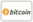Bookcover of Numerical Study for Optimal Control Problems of Parabolic Systems
Booktitle:

# Numerical Study for Optimal Control Problems of Parabolic Systems

## Numerical Methods and Applications

LAP LAMBERT Academic Publishing (2015-02-18 )eligible for voucher
ISBN-13:

### 978-3-8484-3048-2

ISBN-10:
3848430487
EAN:
9783848430482
Book language:
English
Blurb/Shorttext:
The optimal control problems governed by partial differential equations have developed very fast in the last 30 years, and it has brought a promising and vital researching domain to the subject of mathematics. The optimal control problems governed by partial differential equations concern many applications in physics, chemistry, biology, etc., such as materials design, crystal growth, temperature control, petroleum exploitation, and so on. The partial differential equations involved in these problems include elliptic equations, parabolic equations and hyperbolic equations. Our objective of this book, which consists of three chapters, aims to introduce the analytical and numerical solutions of constrained optimal control problems of quasilinear parabolic systems.
Publishing house:
Website:
https://www.lap-publishing.com/
By (author) :
Talaat Abdelhamid, Mahmoud H. Farag
Number of pages:
80
Published on:
2015-02-18
Stock:
Available
Category:
Mathematics
Price:
39.90 €
Keywords:
quasi linear parabolic equation, existence and uniqueness theorems, Adjoint system, Gradient formula, Exterior penalty function - Conjugate gradient algorithm., Optimal Control### Categories